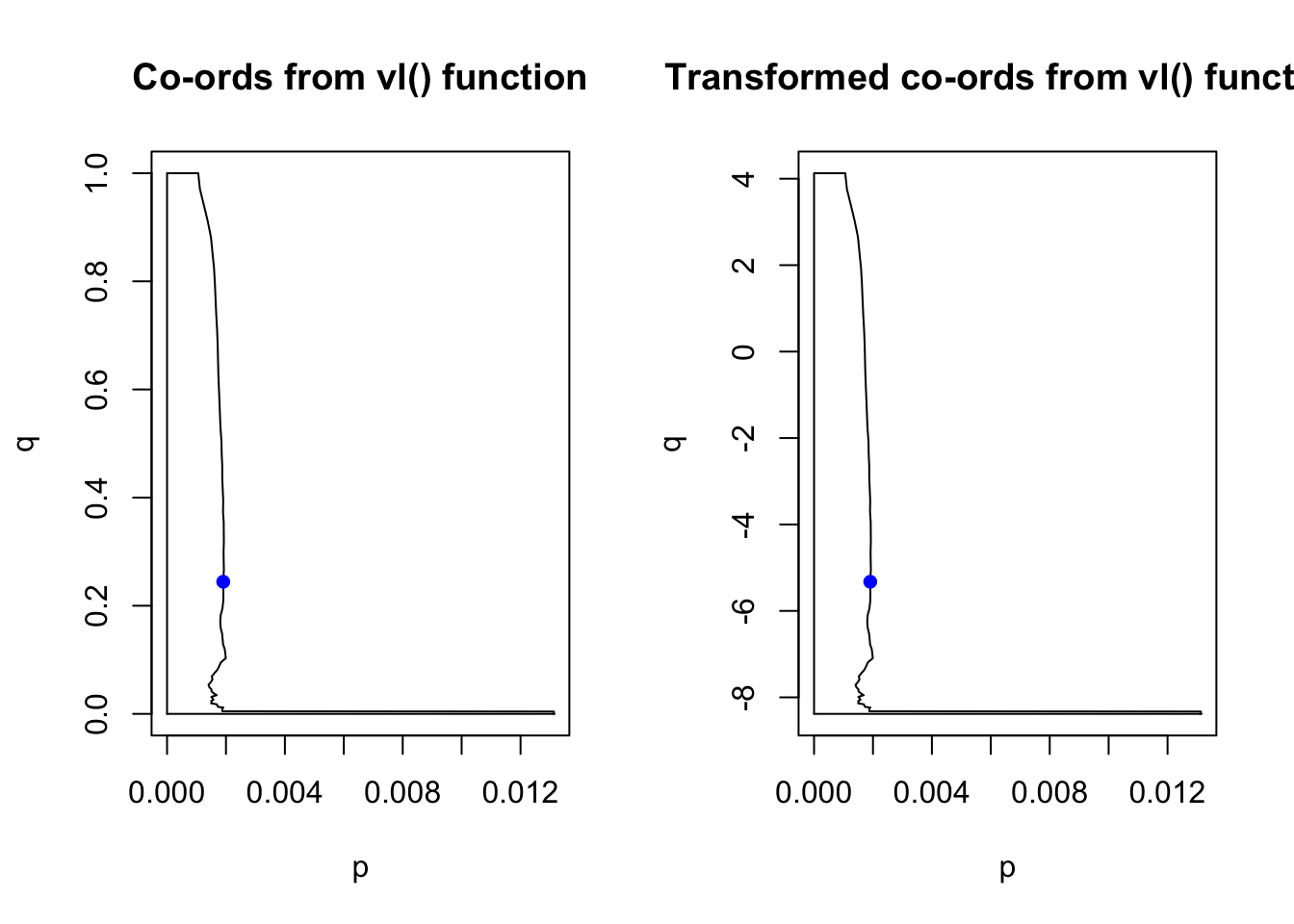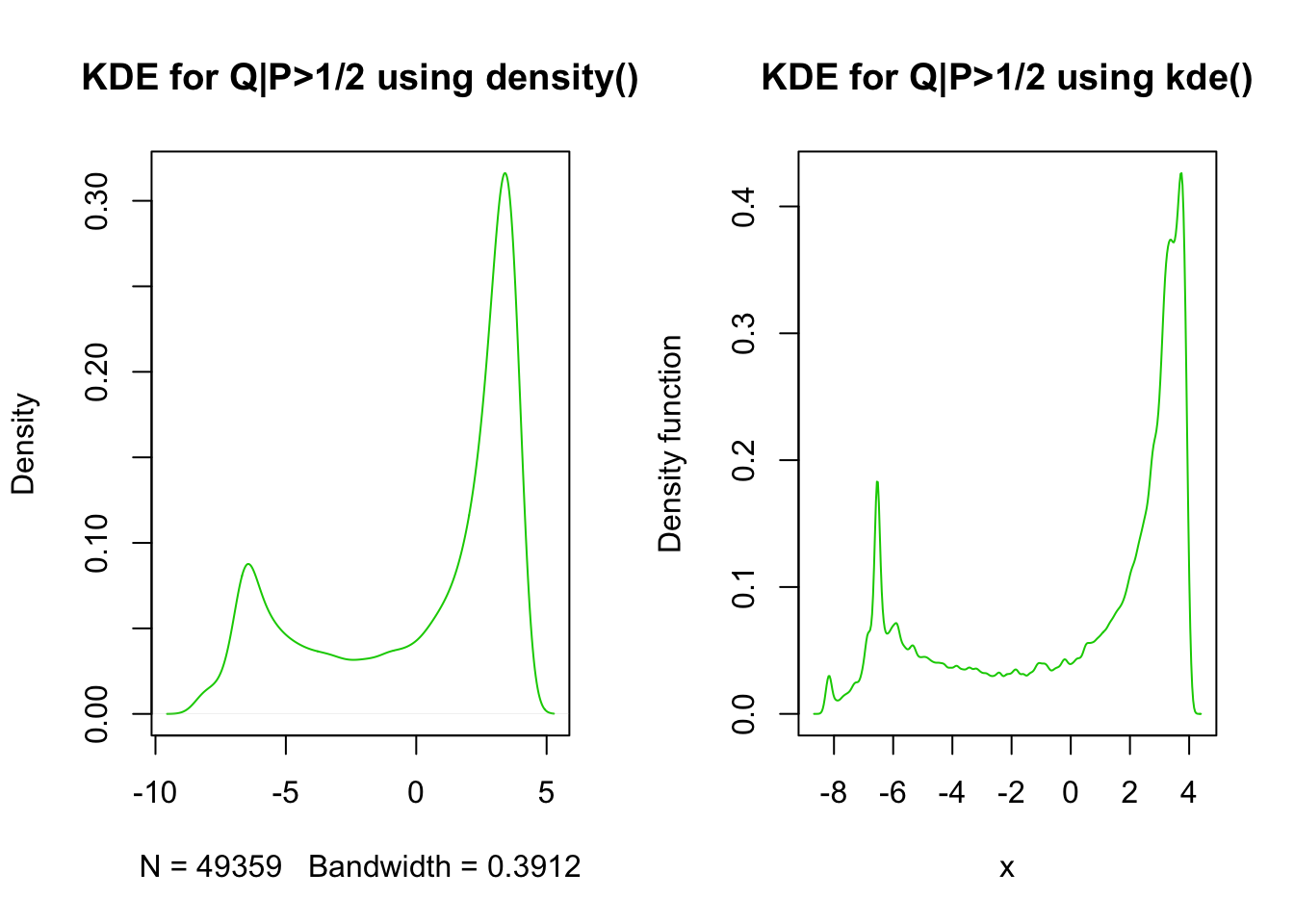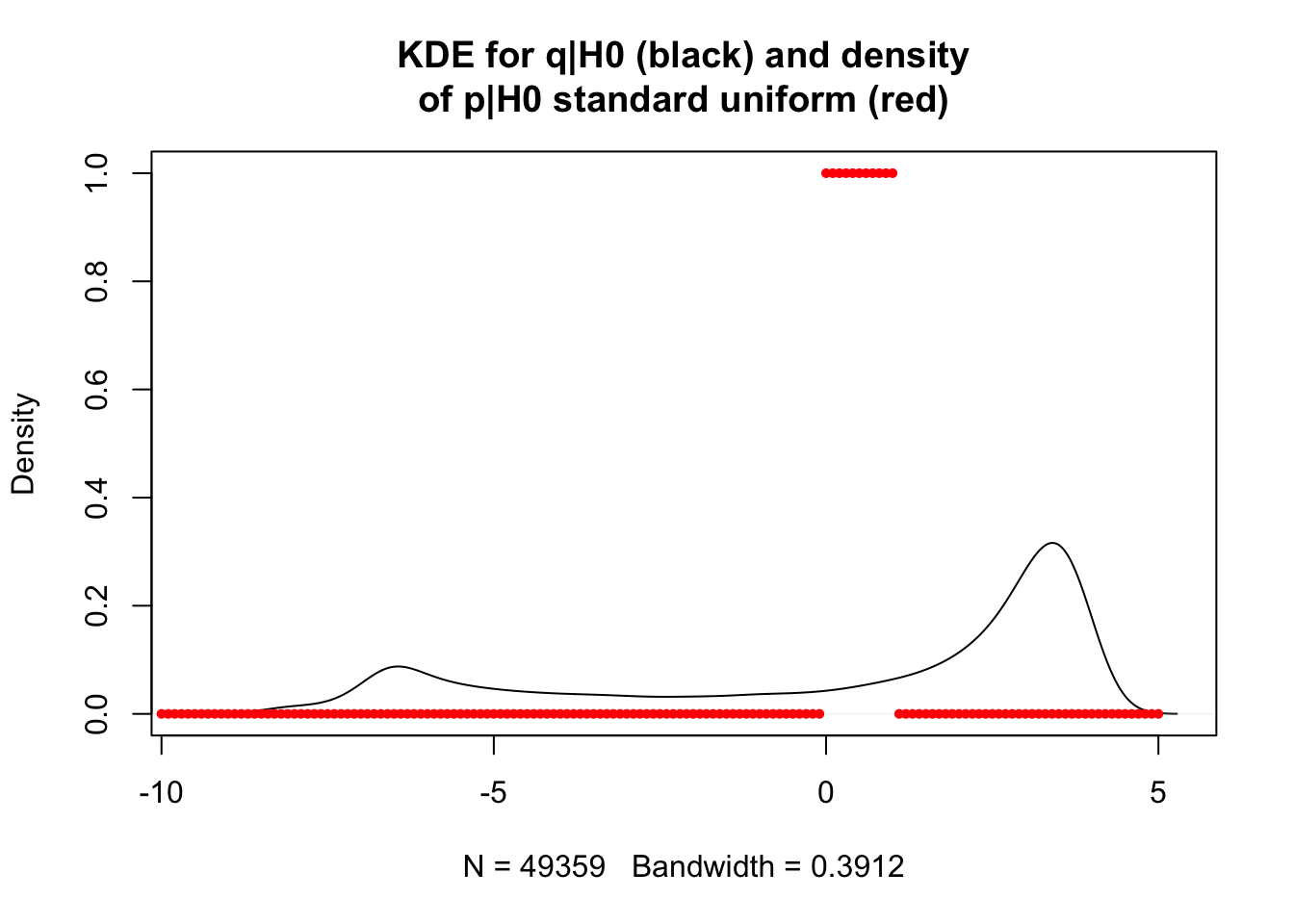## Extending cFDR for continuous annotations

#### 1. Convert q’ back to q range

We consider three variations of the vector of annotations ($$q$$):

1. $$q$$ (original PC) $$\in (-Inf, Inf)$$
2. $$q'$$ (transformed PC) $$\in [0,1]$$
3. $$q''$$ (ranked PC) $$\in [0,1]$$

Last week we settled on using $$q''$$ to deal with the fact that $$q$$ is not a transformed normal as James’ functions assume. We seemed to get away with this because the method ultimately cares about $$Pr(P<p|Q<q)$$ and the functional form for the distribution of Q is convenience.

Our L curves are therefore defined on $$[0,1]\times [0,1]$$ which works for James’ functions, but as I am attempting to rewrite the integration code, I need the L curves to be defined on $$[0,1]\times (-Inf, Inf)$$. As converting back from $$q''$$ to $$q$$ would be hard, I remove the ranking step, calculate the L curves using $$q'$$ (still defined on $$[0,1]\times [0,1]$$) and then convert the $$y$$ co-ordinates of the L curve back to the $$(-Inf, Inf)$$ range.

#' range01
#' convert vector from (-inf, inf) range to [0,1] range
#' @param x vector
#'
#' @return transformed vector
#' @export
range01 <- function(x){(x-min(x))/(max(x)-min(x))}

#' rangeinf
#' convert vector back from [0,1] range to (-inf, inf) range
#' @param x vector in [0,1] range
#' @param s original vector to get max and min from
#'
#' @return vector in (-inf, inf) range
rangeinf <- function(x, s){x*(max(s)-min(s))+min(s)}
final_dataset <- readRDS("final_dataset.RDS")
pca_scale <- prcomp(final_dataset[,c(12:ncol(final_dataset))], center = TRUE, scale = TRUE)
pcs <- pca_scale$x # user would input p in [0,1] and q in (-Inf, Inf) p <- final_dataset$p
q <- pcs[,1]

# we would then transform q to [0,1] range to use vl()
q_trans <- range01(q)

p[which(p<1e-300)] <- 1e-300 # to avoid errors in James' code
q_trans[which(q_trans<1e-300)] <- 1e-300

candidate_indices <- which(p < 0.01) # 9944 of these

chr <- final_dataset$chrom fold <- which(chr == 1) ind <- intersect(candidate_indices, fold) L <- vl(p, q_trans, indices=ind, mode=2, fold=fold) # compute L curves # convert y co-ords back y_range01 <- as.vector(L$y)
y_rangeinf <- rangeinf(y_range01, q)

# consider a single example
p_example <- p[ind]
q_example <- q[ind]
q_trans_example <- q_trans[ind]

par(mfrow=c(1,2))
plot(L$x[1,], L$y, type = "l", main = "Co-ords from vl() function", xlab = "p", ylab = "q")
points(p_example, q_trans_example, col = "blue", pch = 16)

plot(L$x[1,], y_rangeinf, type = 'l', main = "Transformed co-ords from vl() function", xlab = "p", ylab = "q") points(p_example, q_example, col = "blue", pch = 16)#### 2. Function to integrate over We want to integrate the joint distribution given the null. Given our independence assumption, $\begin{equation} f_{p,q'}(p,q|H_0^p)=f_p(p|H_0^p)\times f_{q'}(q|H_0^p) \end{equation}$ where $$p\in[0,1]$$ and $$q'\in[0,1]$$. This is hard to do. However, now that we have transformed the q values back to the $$(-Inf, Inf)$$ range, we have $\begin{equation} f_{p,q}(p,q|H_0^p)=f_p(p|H_0^p)\times f_{q}(q|H_0^p) \end{equation}$ where $$p\in[0,1]$$ and $$q\in(-Inf, Inf)$$. Under the null, we assume that the P values are uniformly distributed, and we can use KDE for the $$q$$ bit (since we are no longer in the $$[0,1]$$ range). Note that James approximated $$Q|P>1/2$$ using a mixture Gaussian because you can’t use KDE when you’re limited to the $$[0,1]$$ range. I can then use the polyCub R package to integrate this continous function over our polygon (the L region given by the L curve). (https://cran.r-project.org/web/packages/polyCub/vignettes/polyCub.html) #### 2a. KDE estimate of $$f(q|H_0^p)$$ I estimate $$f(q|H_0^p)$$ empirically using those $$q$$ values with corresponding $$p>1/2$$ (hopefully representing null cases). For this reason, it is important that our method is used for genomic controlled P values (to ensure they are uniformly distributed under the null). • Should this be approximated using the leave-one-chromosome out method? For now I just use the full dataset to get an estimate. I investigate using both the ks function and the density function for the KDE and decide to settle on the density function as this is more widely used. library(ks) p <- final_dataset$p
q <- pcs[,1]

fqh0_density <- density(q[which(p>1/2)])
fqh0_ks <- kde(q[which(p>1/2)])

par(mfrow=c(1,2))
plot(fqh0_density, col=3, main = "KDE for Q|P>1/2 using density()")
plot(fqh0_ks, col=3, main = "KDE for Q|P>1/2 using kde()")# extract function
fqh0 <- approxfun(fqh0_density$x, fqh0_density$y)

#### 2b. Estimate $$f(p|H_0^p)$$

We assume that this is the standard uniform distribution.

# estimate f_p(p|H_0) with standard uniform
fph0 <- function(x) dunif(x, 0, 1)

#### 2c. Bivariate distribution to integrate

Both the functions are plotted below.# estimate the product of these
# bivariate distribution: f(p,q | H0) = f_p(p | H0) x f_q(q | H0)
# from the polyCub documentation:
# a two-dimensional real-valued function to be integrated over polyregion. As
# its first argument it must take a coordinate matrix, i.e., a numeric matrix with
# two columns, and it must return a numeric vector of length the number of coordinates.
fpqh0 <- function(s){
fph0(s[,1])*fqh0(s[,2])
}

### 3. Integration using polyCub function

The polyCub R package I am using for the integration uses the fact that the integral of a continuously differentiable function $$f(x,y)$$ over a domain $$W\subset {\rm I\!R}^2$$ can be approximated using an $$n$$-point cubature rule of the form:

$\begin{equation} \int \int_W f(x,y)dxdy \approx \sum_{i=1}^n w_i f(x_i, y_i), \end{equation}$

i.e., a weighted sum of function values at an appropriate set of nodes. I am using the general-purpose product Gauss cubature (Sommariva & Vianello, 2007).

library(polyCub)

# make xylist of polygon co-ords
# need points to go anticlockwise to define the hole
new_order <- c(seq(which.max(L$x[1,]), 0, -1),seq(length(L$x[1,]), which.max(L$x[1,])+1, -1)) polygon <- list( list(x = L$x[1,][new_order],
y = y_rangeinf[new_order])
)

# note the polygon is there, just squished on the left and
# it doesn't let me stretch the x axis
plotpolyf(polygon, fpqh0, xlim = c(0,1), ylim = c(-10,10), cuts = 20)